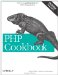# Recipe 23.8. Picking a Random Line from a File

#### 23.8.1. Problem

You want to pick a line at random from a file; for example, you want to display a selection from a file of sayings.

#### 23.8.2. Solution

Use the pc_randomint( ) function shown in Example 23-26, which spreads the selection odds evenly over all lines in a file.

##### Finding a random line of a file

 ` \$line_number = 0; \$fh = fopen('sayings.txt','r') or die(\$php_errormsg); while (! feof(\$fh)) { if (\$s = fgets(\$fh)) { \$line_number++; if (pc_randomint(\$line_number) < 1) { \$line = \$s; } } } fclose(\$fh) or die(\$php_errormsg); ?>`

#### 23.8.3. Discussion

The pc_randomint( ) function computes a random decimal number between 0 and \$max, including 0 but excluding \$max. As each line is read, a line counter is incremented, and pc_randomint( ) generates a random number between 0 and \$line_number. If the number is less than 1, the current line is selected as the randomly chosen line. After all lines have been read, the last line that was selected as the randomly chosen line is left in \$line.

This algorithm neatly ensures that each line in an n line file has a 1/n chance of being chosen without having to store all n lines into memory.

Documentation on mt_rand( ) at http://www.php.net/mt-rand.PHP Cookbook: Solutions and Examples for PHP Programmers
ISBN: 0596101015
EAN: 2147483647
Year: 2006
Pages: 445

Similar book on Amazon